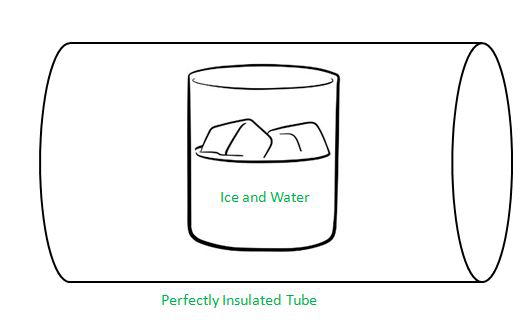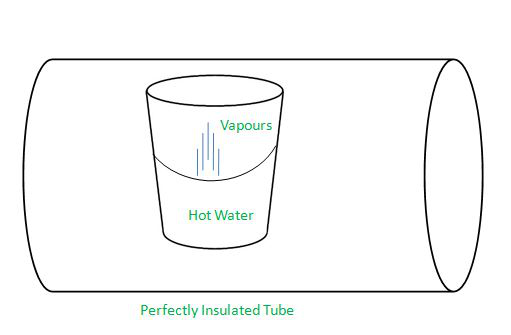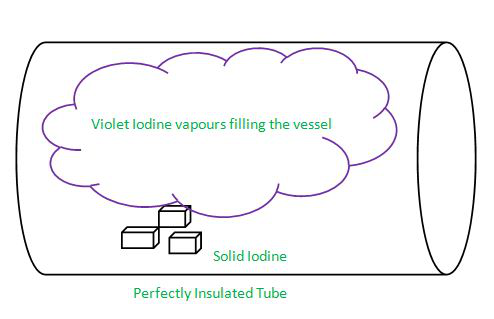GeeksforGeeks App
Open AppBrowser
Continue

## Related Articles

• CBSE Class 11 Chemistry Notes

# What is Equilibrium? – Definition, Types, Laws, Effects

Every chemical reaction wants to attain stability or a state of equilibrium because unstable reactions are of little or no use practically. Equilibrium makes the chemical reaction useful for practical purposes. Equilibrium can be attained not only by chemical processes but also by physical processes. A process can attain an equilibrium state early or late depending on the conditions in which the reaction takes place and on the type of reaction like physical or chemical.

Reactions can be classified into three categories depending on the extent to which they can proceed.

• The first kind of reactions are those which can proceed to their completion means concentrations of products formed are more as only a little or no amount of reactants are left.
• The second kind of reaction is the opposite of the first kind, these reactions proceed to a little extent and most of the concentration of reactants is left and a little amount of product is formed.
• The third kind of reaction is the one in which the concentration of reactants and concentration of products are nearly in equal amounts available.

For Example, there is an equilibrium between oxygen molecules and proteins haemoglobin and only because of this equilibrium transportation of oxygen from lungs to muscles is possible and thus human beings are alive.

### Whatis Equilibrium?

Equilibrium means the state of stability or a state without any physical or chemical disturbance. In the context of chemical equilibrium can be defined as a state when the rate of forward and reverse reactions become equal and no net change is seen in the reaction.

• Reactants: The substances that are used to initiate any chemical reaction are called Reactants.
• Products: The substances that are formed after the end of any chemical reaction are called Products.

Whenever any chemical reaction occurs there is a change in the number of reactants as reactants keep on decreasing and as result products are formed and the number of products increases. After a particular period of time depending on the reaction, there will not be any change in the reactants and products, this state is the state of Equilibrium.

Equilibrium in physical processes is discussed in the below headings.

### Solid-Liquid Equilibrium

Solid-liquid equilibrium is a phase transformation equilibrium in which solid gets converted to liquid and liquid gets converted to solid and when this conversion rate become equal and no visible change is seen in the solid and liquid state then this state is called as Solid-Liquid Equilibrium.

e.g. A glass of ice with some water is kept in a perfectly insulated tube means no energy exchange will happen between the tube and its surroundings. The temperature inside the insulated tube is nearly 0 degrees Celsius.

Observation: The amount of water and ice is not changing with time. Pressure and Temperature are also constant in the insulated tube. This state is Solid-Liquid Equilibrium. But at the molecular level the change is happening as ice is turning into water and water vapours are turned into ice, here the rate of both processes are equal that’s why no visible change is seen.

H2O (solid)  ⇌  H2O (liquid)Solid-Liquid Equilibrium

### Liquid-Vapour Equilibrium

Liquid-Vapour equilibrium is also a phase transformation equilibrium in which liquid gets converted to vapours and vapours gets converted to liquid and when this conversion rate become equal and no visible change is seen in the liquid and vapour state then this state is called as Liquid-Vapour Equilibrium.

e.g. A glass of hot water is kept in a perfectly insulated tube means no energy exchange will happen between the tube and its surroundings. The temperature of the water is its boiling point means 100 degrees Celsius and a pressure of 1.013 bar is maintained inside the insulated tube.

Observation: Hot water is kept so water vapours will evaporate and the pressure inside the tube will increase but after some time pressure will decrease due to the process of condensation. There will be a state at which evaporation and condensation process will occur at equal rates and this state is Liquid-Vapour equilibrium.

H2O (liquid)  ⇌  H2O (vapour)Liquid-Vapour Equilibrium

### Solid-Vapour Equilibrium

Solid-Vapour equilibrium is also a type of phase transformation equilibrium in which solid directly gets converted to vapours without changing into liquid phase and vapours gets converted to solid and when this conversion rate become equal and no visible change is seen in the solid and vapour state then this state is called as Solid-Vapour Equilibrium.

Substances that directly sublimes to vapour state from solid-state are Solid Iodine, Camphor etc.

e.g. Pieces of solid iodine is kept in a perfectly insulated glass tube at room temperature and pressure.

Observation: It is observed that the vessel gets filled up with iodine vapours which are of violet colour and the intensity of colour keeps on increasing for some time after which there is a state after which no change is visible in the solid state of iodine and vapour state of iodine this is the Solid-Vapour equilibrium.

I2 (solid)  ⇌  I2 (vapour)

The above experiment can also be done with Camphor.Solid-Vapour Equilibrium

### Types of Equilibrium

Homogeneous Equilibrium

The equilibrium in which the state of reactants, as well as products, are the same is known as Homogeneous Equilibrium. It means if reactants of a reaction are in the gaseous phase then the products must also be in the gaseous phase, this type of equilibrium is homogeneous in nature.

e.g.

N2(gas) + 3H2(gas) ⇌  2NH3(gas)

Here the reactants mean Nitrogen and Hydrogen both are in the gaseous phase and the product which is ammonia is also in the gaseous phase so this is a homogeneous equilibrium.

Heterogeneous Equilibrium

The equilibrium in which the state of reactants and state of products are different then is known as Heterogeneous Equilibrium. It means if reactants of a reaction are in the gaseous phase then the products can be in some other phase, this type of equilibrium is heterogeneous in nature.

e.g.

H2O(liquid) ⇌ H2O(gas)

The above reaction is the phase transformation reaction in which water is converted from liquid phase to gaseous phase and this type of equilibrium is known as heterogeneous equilibrium.

### Law of Chemical Equilibrium

To understand the law of chemical equilibrium let’s take a reversible reaction that is in an equilibrium state.

R1 + R2  ⇌  P1 + P2

R1 and R2 are reactants and P1 and P2 are products formed in a chemical reaction.

According to different researches and experiments, it has been found that reactants and products of a chemical reaction that is in equilibrium are related by an Equilibrium reaction and this is firstly proposed by Cato Maximillian Guldberg and Peter Waage in 1864.

Kc = ([P1][P2])/([R1][R2])

Kc is known as the Equilibrium Constant and the equation on the other side is called Equilibrium constant expression.

The above chemical reaction is just for reference purposes as practical chemical reactions are balanced also means the above reaction should have stoichiometric coefficients (these are generally the numbers which are multiplied on both side reactants side as well as products side.) So the balanced reaction is

aR1 + bR2  ⇌  cP1 + dP2

where a, b, c and d are stoichiometric coefficients for balancing the chemical equation.

Now Kc can be rewritten as:

Kc = ([P1]c[P2]d)/([R1]a[R2]b)

At a given temperature, multiplying the products of a balanced chemical reaction along with their stoichiometric coefficient given as powers and then dividing the equation by-product of reactants along with their stoichiometric coefficient given as powers will yield a fixed value. This is known as the Law of Chemical Equilibrium.

Equilibrium Constant in Gaseous Systems

It is easier to represent Equilibrium Constant (Kc) in terms of pressure as Kp when reactions are in a gaseous state. So gas equation is given as,

pV = nRT

p = n/V(RT)

Here p is the pressure which has unit pascal and V is Volume in cubic meter and T is the temperature in Kelvin, n is number of moles of gas and R is constant which has a value of 0.0831 bar litre /mol Kelvin.

Let’s understand by a reaction as:

N2 + 3H2  ⇌  2NH

In this reaction reactants and products are in gaseous phase so lets find Kp.

Kp =  (pNH3)2/(pN2)(pH2)

= (NH3)2(RT)2/[N2RT][(H2)3(RT)3]

= Kc(RT)-2

It is clear that Kp and Kc are related now general equation can be derived as,

Kp = Kc(RT)∇n

Δn represents (Total number of moles of on products side) – (total number of moles of reactants side) in the balanced chemical equation.

### Factor affecting Equilibrium

The effect of different factors on equilibrium can be understood by Le Chatelier’s principle. It states that a change in any of the factors that determine the equilibrium conditions of a system will cause the system to change in such a manner so as to reduce or counteract the effect of the change. This is applicable to all physical and chemical equilibria.

Concentration

• When reactant or product is added then according to Le Chatelier’s principle the reaction will proceed in the direction in which the added reactant or product will get consumed.
• When reactant or product is removed then according to Le Chatelier’s principle the reaction will proceed in the direction in which the removed reactant or product will be replenished.

Pressure

• Pressure change does not affect equilibrium in the case of solid and liquid phase reactions as the concentration of solids and liquids does not depends on pressure.
• In the case of gases if pressure is increased then the direction of reaction will become opposite and equilibrium will be shifted in the backward direction.

Temperature

• In case of exothermic reactions (reactions that liberate heat and energy when proceeds to completion) the equilibrium constant decreases when the temperature is raised.
• In the case of endothermic reactions (reactions that consumes heat and energy when proceeds to completion), the equilibrium constant increases as temperature are increased.

Effect of Inert Gas

• The addition of inert gas to a reaction does not affect equilibrium provided volume is kept constant because if the volume is constant then inert gas will not be able to change partial pressures and hence equilibrium will be undisturbed.
• In case if volume is not constant then the inert gas will change the reaction quotient only if gas is taking part in the reaction either as reactant or product.

### Sample Questions

Question 1: Find the equilibrium constant for the given reaction N2 (gas)+ 3H2 (gas)  ⇌  2NH3 (gas) at 500 K temperature, when concentrations are N2 =2×10-2 M, H2 = 4×10-2 M and NH3 = 1×10-2 M.

Use Equilibrium constant reaction which is,

Kc = ([P1]c[P2]d)/([R1]a[R2]b)

Therefore,

Kc = ([NH3]2)/([N2][H2]3)

= (1×10-2)2/(2×10-2)(4×10-2)3

= 0.00781 × 104

= 78.1

Question 2: Find the equilibrium constant for the given reaction PCl5  ⇌ PCl3 + Cl2 at 500 K temperature, when concentrations are PCl5 = 1.5 M, PCl3 = 2 M and Cl2 = 2 M.

Kc = [PCl3][Cl2]/[PCl5]

= /[1.5]

= 2.66

Question 3: The equilibrium constant for the given reaction: N2 + O2 ⇌ 2NO is 2×10-2 at 300 K.

In the presence of a catalyst the equilibrium attains ten times faster, find the equilibrium constant in presence of a catalyst.

In such type of questions try to understand the question first so as catalysts are the materials that are used to speed up the chemical processes. If a process is completed faster means the rate of reactant decreasing and the rate of product formation is also faster so overall it will not affect the equilibrium constant. Hence equilibrium constant will remain unchanged.

Question 4: Find the equilibrium constant for the given reaction:

N2 + O2  ⇌ 2NO at 800 K temperature,

when concentrations are NO = 1×10-2 M, O2 = 2×10-2 M and N2 = 2×10-2 M.

Kc can be calculated by using formula:

Kc = [NO]2/[N2][O2]

= [1×10-2]2/[2×10-2][2×10-2]

= 0.25

Question 5: Write two factors on which equilibrium constant (Kc) depends.